NCERT Exemplar Class 11 Maths Chapter 8 Binomial Theorem are part of NCERT Exemplar Class 11 Maths. Here we have given NCERT Exemplar Class 11 Maths Chapter 8 Binomial Theorem.

## NCERT Exemplar Class 11 Maths Chapter 8 Binomial Theorem

Q1. Find the term independent of x, where x≠0, in the expansion of $${ \left( \frac { 3{ x }^{ 2 } }{ 2 } -\quad \frac { 1 }{ 3x } \right) }^{ 15 }$$Q2. If the term free from x is the expansion of  $${ \left( \sqrt { x } -\frac { k }{ { x }^{ 2 } } \right) }^{ 10 }$$ is 405, then find the value of k.

Sol: Given expansion is $${ \left( \sqrt { x } -\frac { k }{ { x }^{ 2 } } \right) }^{ 10 }$$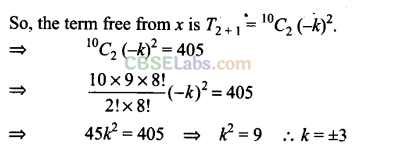Q3. Find the coefficient of x in the expansion of (1 – 3x + 1x2)( 1 -x)16.

Sol: (1 – 3x + 1x2)( 1 -x)16Q4. Find the term independent of x in the expansion of $${ \left( 3x-\frac { 2 }{ { x }^{ 2 } } \right) }^{ 15 }$$

Sol: Given Expression $${ \left( 3x-\frac { 2 }{ { x }^{ 2 } } \right) }^{ 15 }$$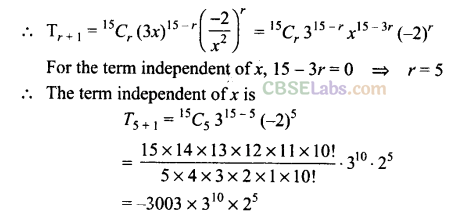Q5. Find the middle term (terms) in the expansion of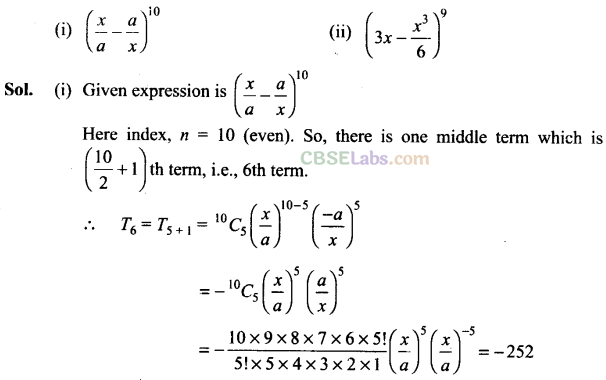Q6. Find the coefficient of x15 in the expansion of $${ \left( x-{ x }^{ 2 }\quad \right) }^{ 10 }$$

Sol: Given expression is   $${ \left( x-{ x }^{ 2 }\quad \right) }^{ 10 }$$Q7. Find the coefficient of $$\frac { 1 }{ { x }^{ 17 } }$$ in the expansion of $${ \left( { x }^{ 4 }-\frac { 1 }{ { x }^{ 3 } } \quad \right) }^{ 15 }$$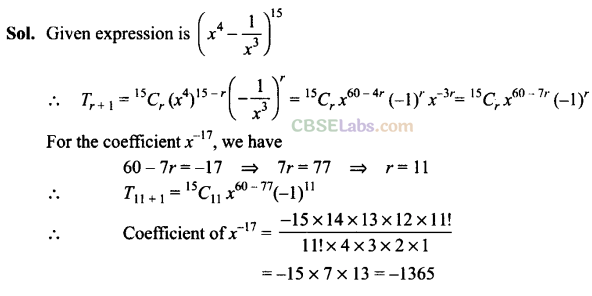Q8. Find the sixth term of the expansion (y1/2 + x1/3)n, if the binomial coefficient of the third term from the end is 45.

>Q9. Find the value of r, if the coefficients of (2r + 4)th and (r – 2)th terms in the expansion of (1 + x)18 are equal.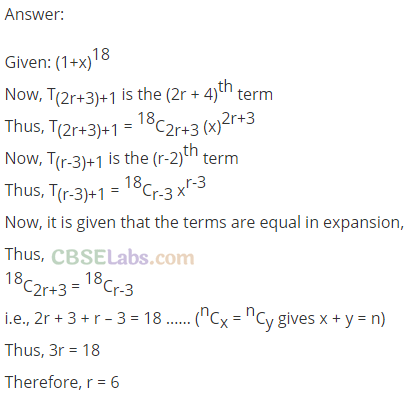Q10. If the coefficient of second, third and fourth terms in the expansion of (1 + x)2” are in A.P., then show that 2n2 – 9n + 7 = 0.Q11. Find the coefficient of x4 in the expansion of (1 + x + x2 + x3)11.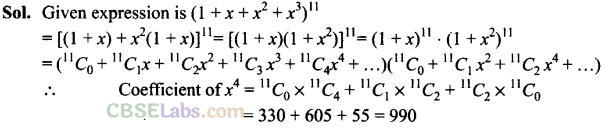Q12. If p is a real number and the middle term in the expansion $${ \left( \frac { p }{ 2 } +2\quad \right) }^{ 8 }$$ is 1120, then find the value of p.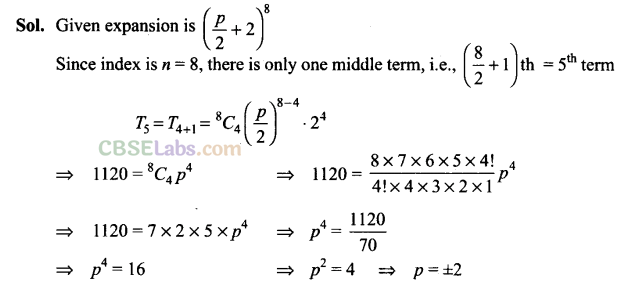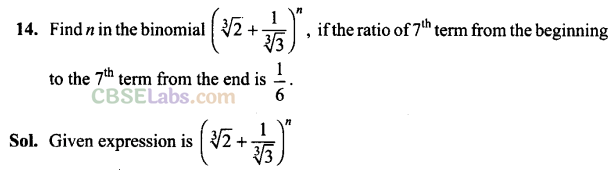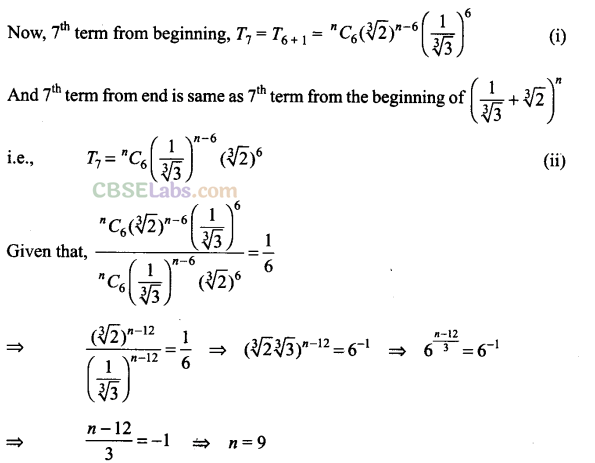Q15. In the expansion of (x + a)n, if the sum of odd term is denoted by 0 and the sum of even term by Then, prove that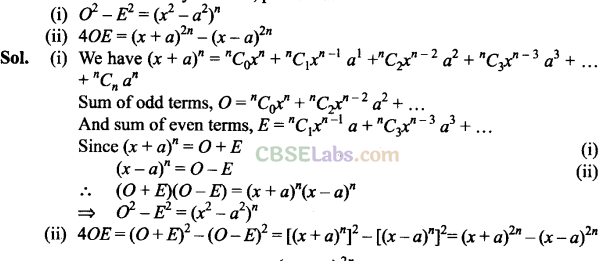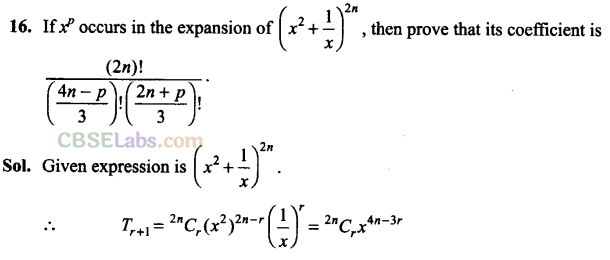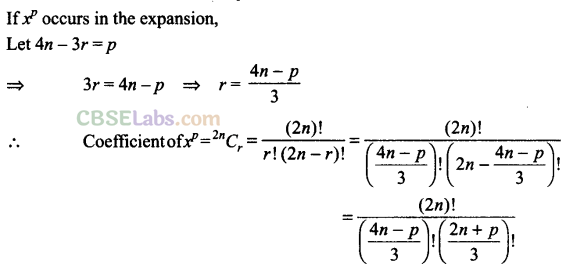Q17. Find the term independent ofx in the expansion of (1 +x + 2x3)$${ \left( \frac { 3 }{ 2 } { x }^{ 2 }-\frac { 1 }{ 3x } \quad \quad \right) }^{ 9 }$$Objective Type Questions

Q18. The total number of terms in the expansion of (x + a)100 + (x – a)100 after simplification is

(a) 50
(b) 202
(c) 51
(d) none of these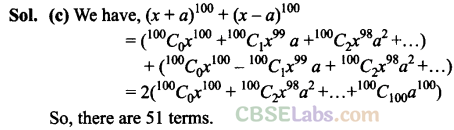Q19. If the integers r > 1, n > 2 and coefficients of (3r)th and (r + 2)nd terms in the binomial expansion of (1 + x)2n are equal, then
(a) n = 2r
(b) n = 3r
(c) n = 2r + 1
(d) none of these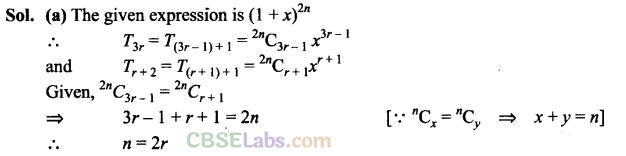Q20. The two successive terms in the expansion of (1 + x)24 whose coefficients are in the ratio 1 : 4 are
(a) 3rd and 4th

(b) 4th and 5th
(c) 5th and 6th
(d) 6th and 7th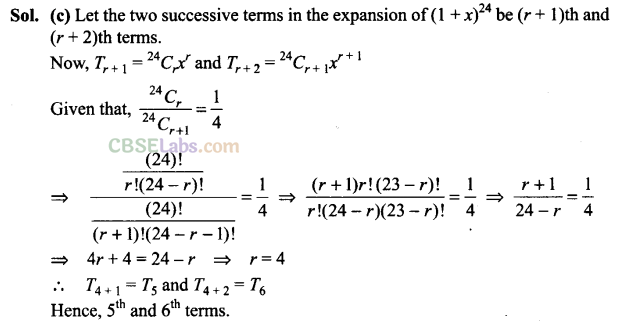Q21. The coefficients of xn in the expansion of (1 + x)2n and (1 + x)2n ~1 are in the ratio
(a) 1 : 2
(b) 1 : 3
(c) 3 : 1
(d) 2:1Q22. If the coefficients of 2nd, 3rd and the 4th terms in the expansion of (1 + x)n are in A.P., then the value of n is
(a) 2

(b) 7
(c) 11
(d) 14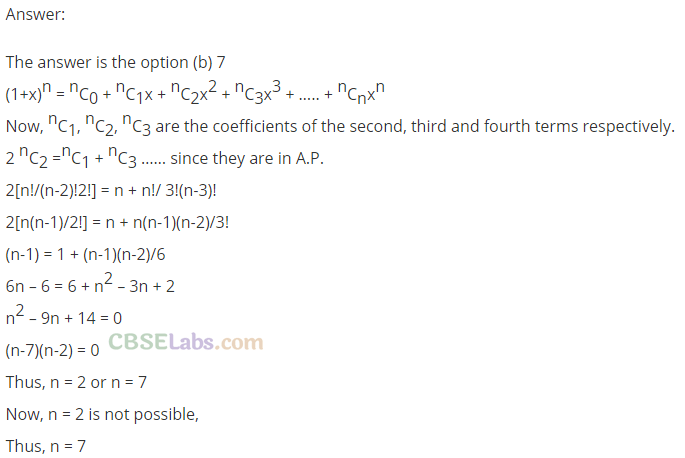Q23. If A and B are coefficients of  xn   in the expansions of (1 + x)2n and (1 + x)2n1  respectively, then A/B  equals to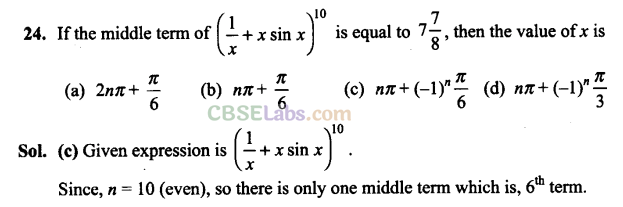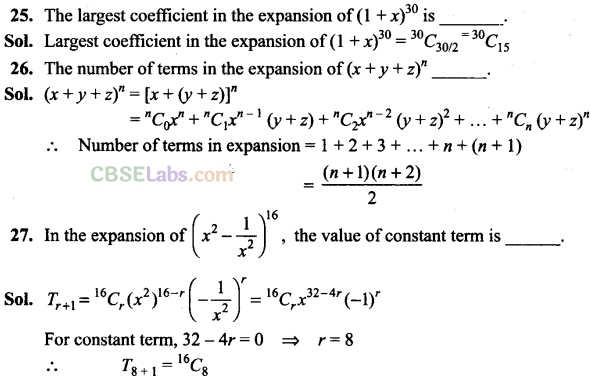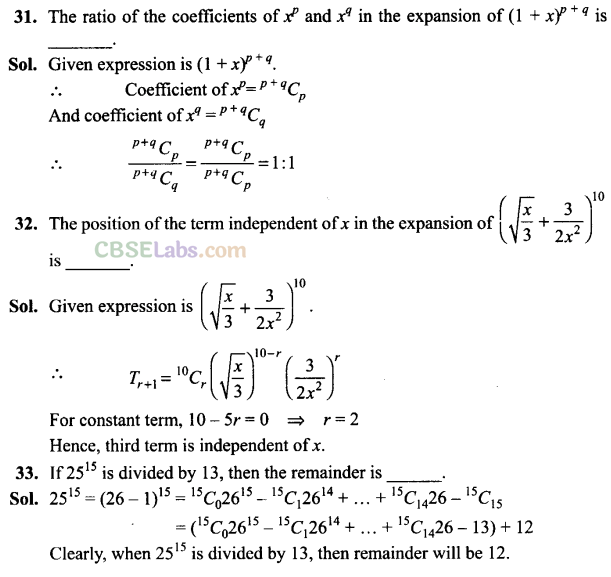We hope the NCERT Exemplar Class 11 Maths Chapter 8 Binomial Theorem help you. If you have any query regarding NCERT Exemplar Class 11 Maths Chapter 8 Binomial Theorem, drop a comment below and we will get back to you at the earliest.